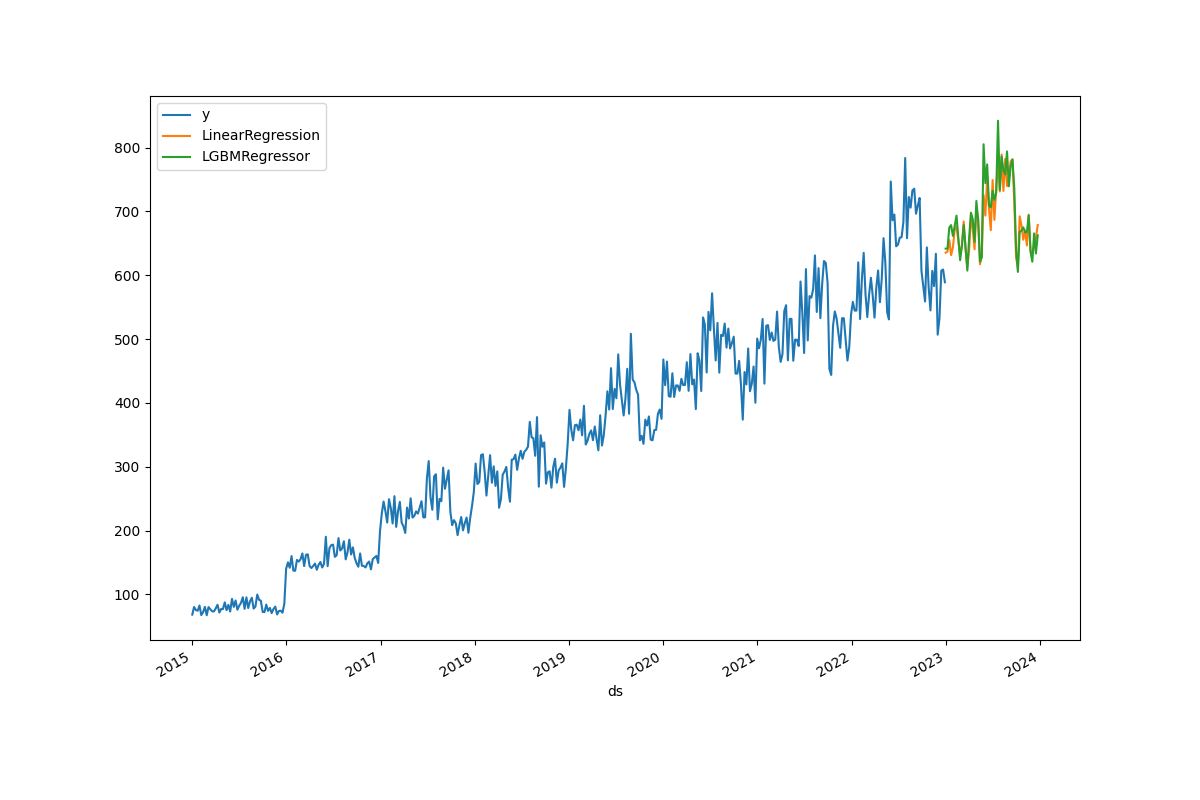07/05 15:36

# 介绍

TDengine™ 是一种开源的云原生时序数据库（ Time Series Database，TSDB），专为物联网（IoT）、连接汽车和工业物联网进行了优化。它能够高效地实时摄取、处理和监控一天内由数十亿个传感器和数据收集器产生的PB级别的数据。

# 演示

## 步骤2：克隆源代码

git clone https://github.com/sangshuduo/td-forecasting

## 步骤3：安装所需的Python软件包

# if you are using Ubuntu 20.04 Linux
sudo apt install python3-pyqt5

# 如果 PyQT5 运行失败，可能需要安装
sudo apt-get install libxcb-xinerama0

python3 -m pip install -r requirements.txt

## 步骤4：模拟一些数据

python3 mockdata.py

## 步骤5：预测明年的数据

python3 forecast.py

## 输出结果# 工作原理介绍

## mockdata.py

...
def insert_rec_per_month(conn, db_name, table_name, year, month):
increment = (year - 2014) * 1.1
base = int(10 * increment)
if month < 10 and month > 5:
factor = 10
else:
factor = 8
for day in range(1, monthrange(year, month) + 1):
num = base * randint(5, factor) + randint(0, factor)
sql = f"INSERT INTO {db_name}.{table_name} VALUES ('{year}-{month}-{day} 00:00:00.000', {num})"
try:
conn.execute(sql)
except Exception as e:
print(f"command: {sql}")
print(e)
...

## forecast.py

### 步骤 1：导入预测需要的 Python 模块

import argparse

import lightgbm as lgb
import matplotlib.pyplot as plt
import mlforecast
import pandas as pd
from mlforecast.target_transforms import Differences
from sklearn.linear_model import LinearRegression
from sqlalchemy import create_engine, text
...

• Lightgbm 是一个 Python 模块，支持 LightGBM 算法，它是一个使用基于树的学习算法的梯度提升框架。
• Matplotlib 是最流行的 Python 模块之一，用于可视化。
• Mlforecast 是一个框架，用于使用机器学习模型执行时间序列预测。
• Pandas 是支持数据操作的最流行的模块。
• Sklearn 是一个支持流行的数据科学/机器学习算法的模块。
• SQLAlchemy 是 Python SQL 工具包和对象关系映射器，它为应用程序开发人员提供了完整的 SQL 功能和灵活性。

### 步骤 2：连接到 TDengine 并查询数据

...
engine = create_engine("taos://root:taosdata@localhost:6030/power")
conn = engine.connect()
print("Connected to the TDengine ...")
text("select _wstart as ds, avg(num) as y from power.meters interval(1w)"), conn
)
conn.close()
...
TDengine Python 连接器提供符合 Python 数据库 API 规范 v2.0（PEP 249）的接口。DBAPI 是“Python 数据库 API 规范”的缩写。这是 Python 中广泛使用的规范，用于定义所有数据库连接包的常用用法模式。DBAPI 是一个“低级别”API，通常是 Python 应用程序中用于与数据库交互的最低级别系统。SQLAlchemy 的方言系统是围绕DBAPI 的操作构建的，提供适用于特定数据库引擎的特定 DBAPI 的各个方言类。

### 步骤 3：预测

...
df.insert(0, column="unique_id", value="unique_id")

print("Forecasting ...")
forecast = mlforecast.MLForecast(
models=[LinearRegression(), lgb.LGBMRegressor()],
freq="W",
lags=,
target_transforms=[Differences()],
)
forecast.fit(df)

predicts = forecast.predict(52)

pd.concat([df, predicts]).set_index("ds").plot(figsize=(12, 8))
...

### 步骤 4: Show up or dump to file

...
if args.dump:
plt.savefig(args.dump)
else:
plt.show()
Python 代码提供了一个参数"--dump"，让用户可以决定将结果转储到图片中进行后续处理，或者立即在屏幕上显示结果。

# 总结0 评论
0 收藏
0